# GSEB Solutions Class 7 Maths Chapter 15 Visualising Solid Shapes Ex 15.2

Gujarat Board GSEB Textbook Solutions Class 7 Maths Chapter 15 Visualising Solid Shapes Ex 15.2 Textbook Questions and Answers.

## Gujarat Board Textbook Solutions Class 7 Maths Chapter 15 Visualising Solid Shapes Ex 15.2Question 1.
Use isometric dot paper and make an isometric sketch for each one of the given shapes: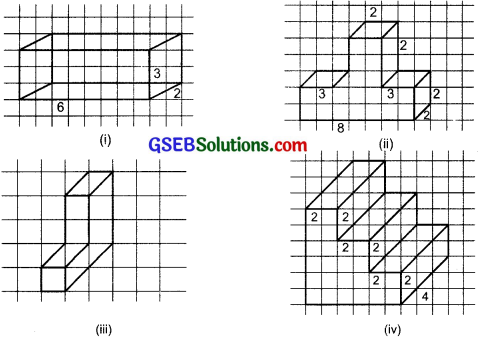Solution: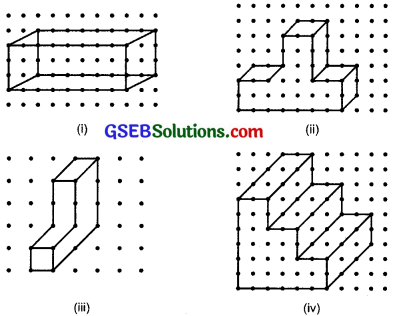Question 2.
The dimensions of a cuboid are 5 cm, 3 cm, and 2 cm. Draw three different isometric sketches of this cuboid.
Solution: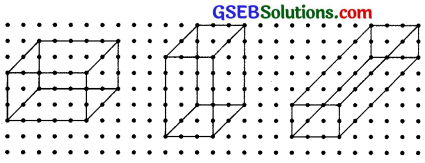Question 3.
Three cubes each with 2 cm edge are placed side by side to form a cuboid. Sketch an oblique or isometric sketch of this cuboid.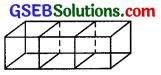Solution:
The adjoining figure is an isometric sketch of a cuboid formed by placing three cubes each of 2 cm edge side by side.Question 4.
Make an oblique sketch for each one of the t given isometric shapes:
Solution:
By taking a squared paper, do it yourself.

Question 5.
Give (i) an oblique sketch and (ii) an isometric sketch for each of the following: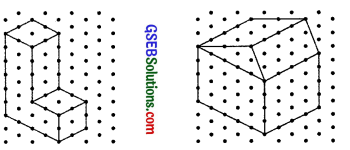(a) A cuboid of dimensions 5 cm, 3 cm and 2 cm. (Is your sketch unique?)
(b) A cube with an edge 4 cm long. An isometric sheet is attached at the end of the book. You could try to make on it some cubes or cuboids of dimensions specified by your friend.
Solution:
Take a squared paper for oblique sketch and an isometric sheet for isometric sketch and then draw –
(a) the cuboid of dimensions 5 cm, 3 cm and 2 cm and
(b) the cube of edge 4 cm. The sketch of this cuboid is not unique